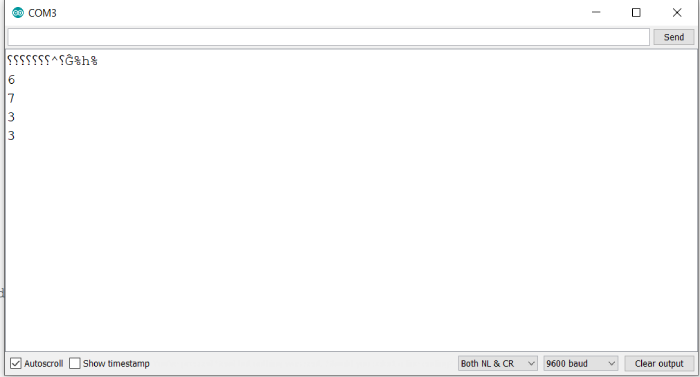# Clear/Set a specific bit of a number in Arduino

When you delve into advanced firmware, you deal with a lot of registers, whose specific bits need to be set or cleared depending on your use case. Arduino has inbuild functions to do that.

## Syntax

bitSet(x, index)

and,

bitClear(x, index)

Where x is the number whose bit has to be set/ cleared and index is the position of the bit (0 for least significant or right-most bit). This function makes changes in the number x in place, and also returns the updated value of x.

Note that setting a bit means setting its value to 1, and clearing it means setting its value to 0.

## Example

The following example illustrates the use of these functions −

void setup() {
// put your setup code here, to run once:
Serial.begin(9600);
Serial.println();
int x = 6;

Serial.println(x);
bitSet(x,0);
Serial.println(x);
bitClear(x,2);
Serial.println(x);
bitClear(x,3);
Serial.println(x);
}

void loop() {
// put your main code here, to run repeatedly:
}

## Output

The Serial Monitor output is shown below −As you can see, we start off with the number 6 (0b0110).

We then set its 0th bit, yielding (0b0111), which corresponds to 7.

Then, we clear its 2nd bit, yielding (0b0011), which corresponds to 3.

We then clear its 3rd bit, which was already 0. Thus, we again get (0b0011), which corresponds to 3.

The Serial Monitor output shows these numbers in the exact sequence we just described.